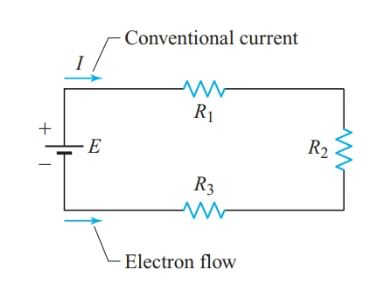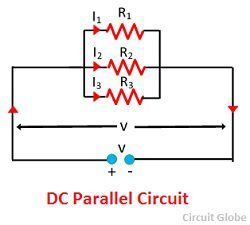# Simple Definition Of Series And Parallel Circuits

By | October 31, 2022

When it comes to constructing electronic circuits, two of the most common terms you'll come across are series and parallel circuits. But, what do these terms actually mean? In this article, we'll break down what a series circuit and a parallel circuit are, as well as their differences, to give you a better understanding of how they work and their advantages in electronic applications.

A series circuit is a type of electrical circuit in which components are connected in a chain, one after another. This means that current (or electricity) only has one path to follow, usually from a power source such as a battery, to each component and back to the power source. This is important to note because in a series circuit, each component is connected directly to the next in the chain, so if one component fails, then all others connected will also be affected. One of the most common examples of a series circuit is a Christmas tree light string, where if one light goes out, then the whole string of lights won’t work.

Parallel circuits, on the other hand, are circuits in which components are connected side-by-side instead of in a chain. This means that the current (or electricity) can split up into multiple paths and go through each component at the same time. This is advantageous because if one component fails, the other components in the circuit can still work, unlike in a series circuit. An example of a parallel circuit is a house wiring system, where the electricity can take different branches to power different lights and appliances throughout the house.

The main difference between a series and a parallel circuit is that in a series circuit, the current flows through only one path, while in a parallel circuit, the current can take multiple paths. Additionally, series circuits are more susceptible to failure if one component fails, while parallel circuits can still work even if one component fails.

To summarize, series circuits are circuits in which components are connected in a chain, so the current can only flow through one path. Parallel circuits, on the other hand, are circuits in which components are connected side-by-side, allowing the current to split up into multiple paths. Knowing the differences between these two types of circuits is important for understanding how they work and their advantages in electronic applications.Basic Electronics On Twitter Parallel Circuit Vs Series Learning Engineering Electricalandelectronicengineering Https T Co Nplj3xrss2Parallel Circuit Stickman PhysicsEquivalent Resistance What Is It How To Find Electrical4uWhat Is A Series Parallel Circuit Combination Circuits Electronics TextbookDifference Between Series And Parallel Circuits With Its Practical Applications In Real LifeTypes Of Circuits HowstuffworksParallel Circuit Definition Example LinquipElectrical Electronic Series CircuitsParallel Circuit Definition Examples Electrical AcademiaSeries Circuit Definition Examples Resistors In Electrical A2zWhat S The Easiest Way To Explain Difference Between Parallel And Series Wiring QuoraWhat Is A Dc Circuit Definition Types GlobeSeries Circuit Overview Examples What Is A Lesson Transcript Study ComSeries And Parallel CircuitsDifference Between Series And Parallel Circuit With Comparison Chart GlobeElectrical Electronic Series CircuitsRlc Circuit Analysis Series And Parallel Clearly Explained Electrical4uElectrical Electronic Series CircuitsWhat Is Series Circuit Definition Calculation Linquip